# NCERT Solutions for Class 9 Science Chapter 3 – Atoms and Molecules

NCERT Solutions for Class 9 Science Chapter 3 – Atoms and Molecules, contains solutions to various questions in Exercises for Chapter 3. Atoms and Molecules Class 9 NCERT Solutions have been explained in a simple and easy to understand manner. We are providing all subjects which can be accessed by clicking here.

## Download NCERT Solutions for Class 9 Science Chapter 3 – Atoms and Molecules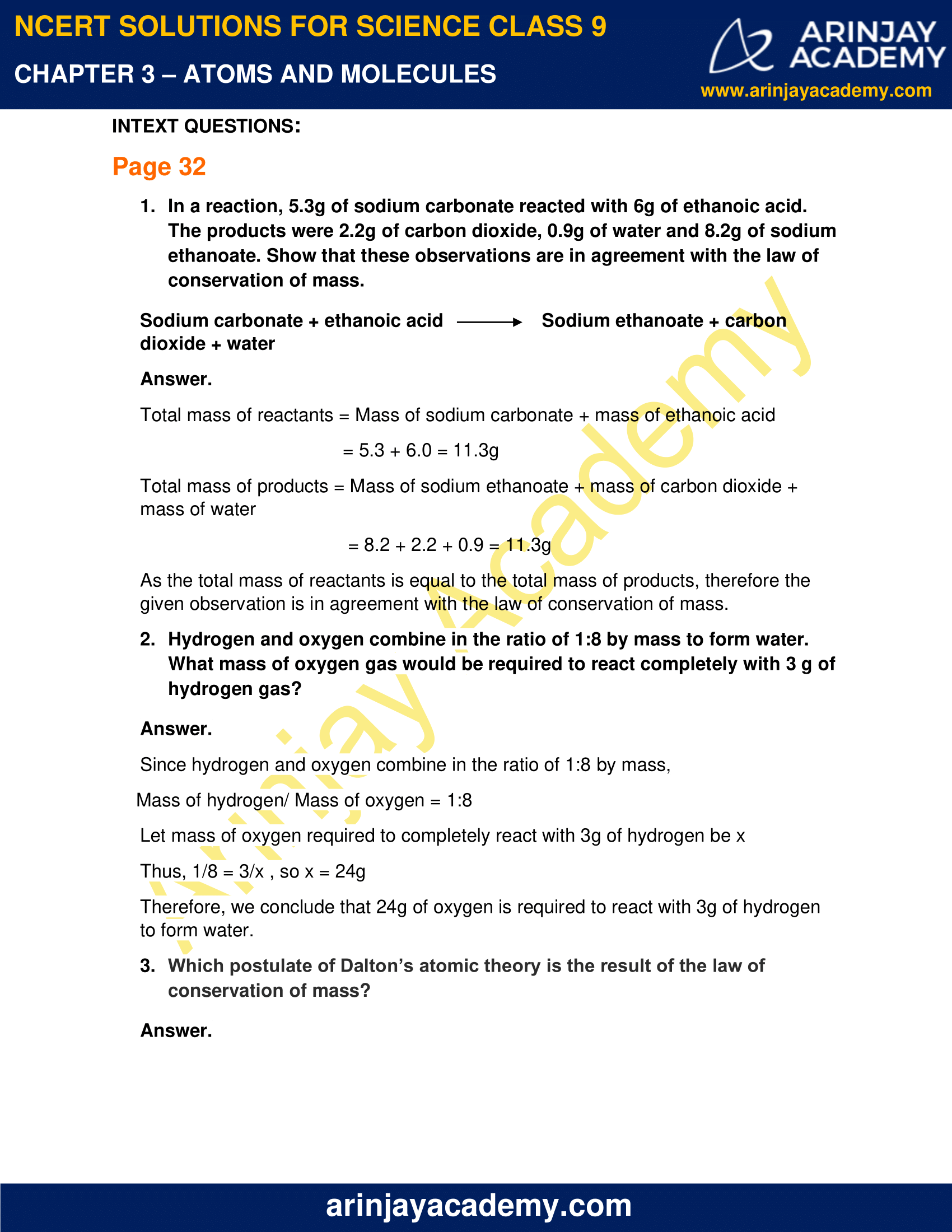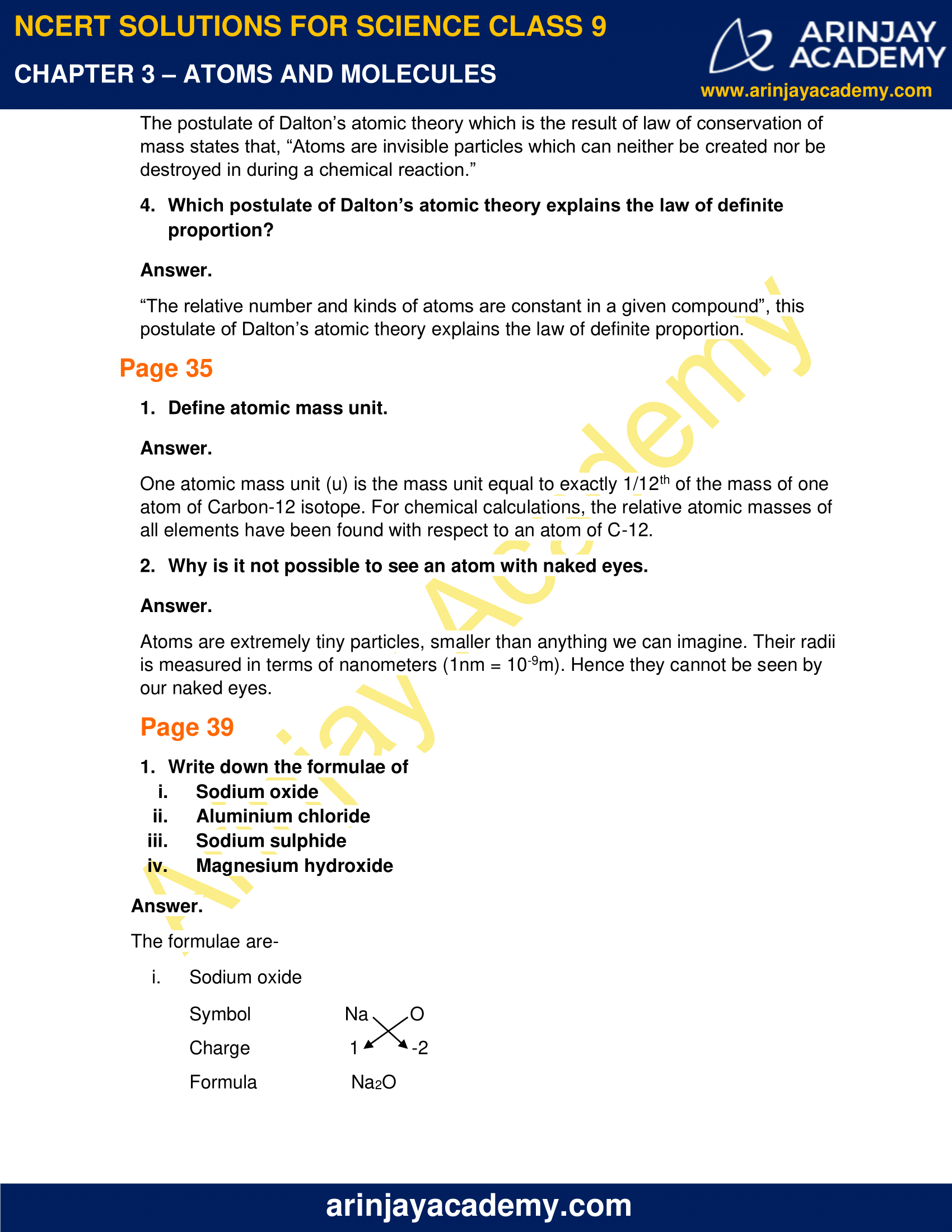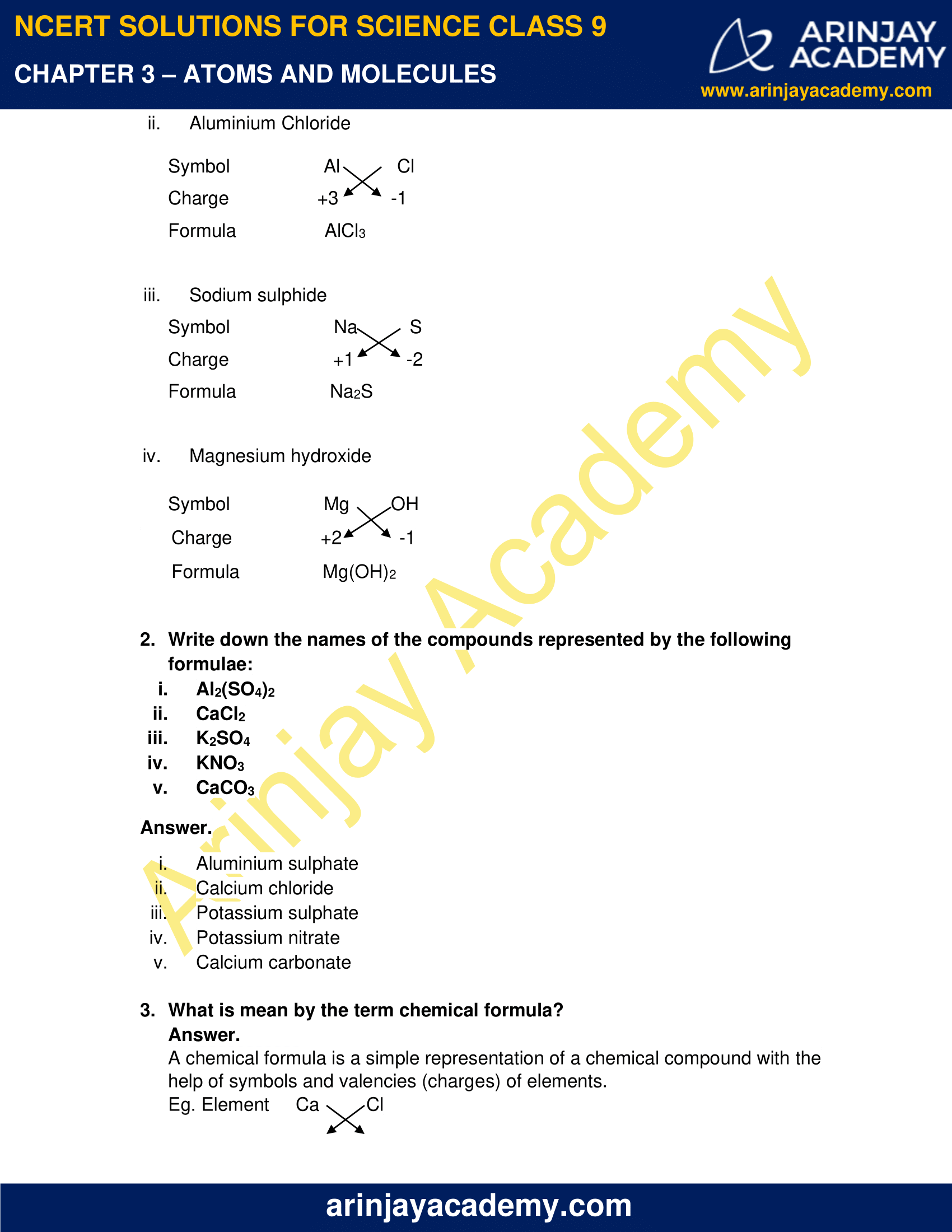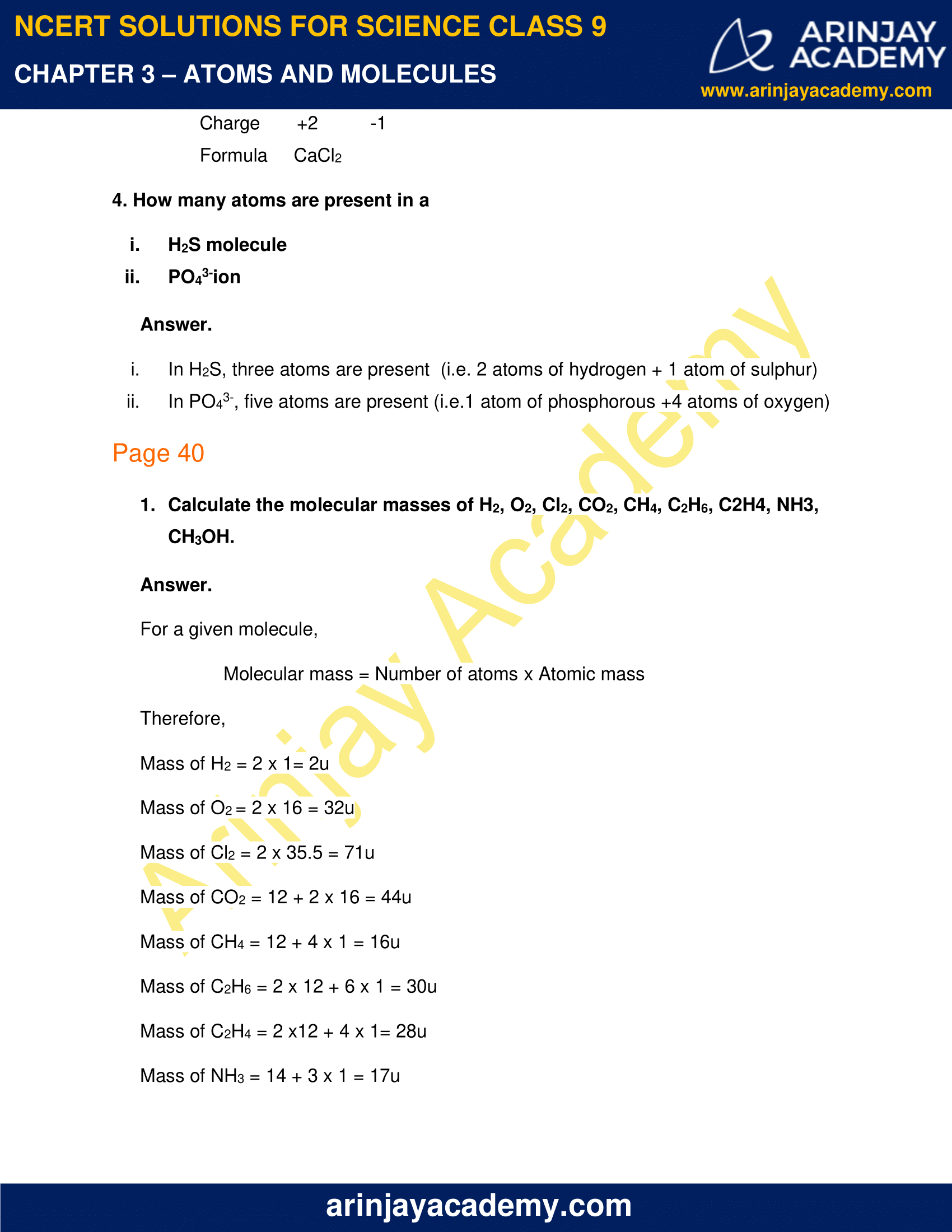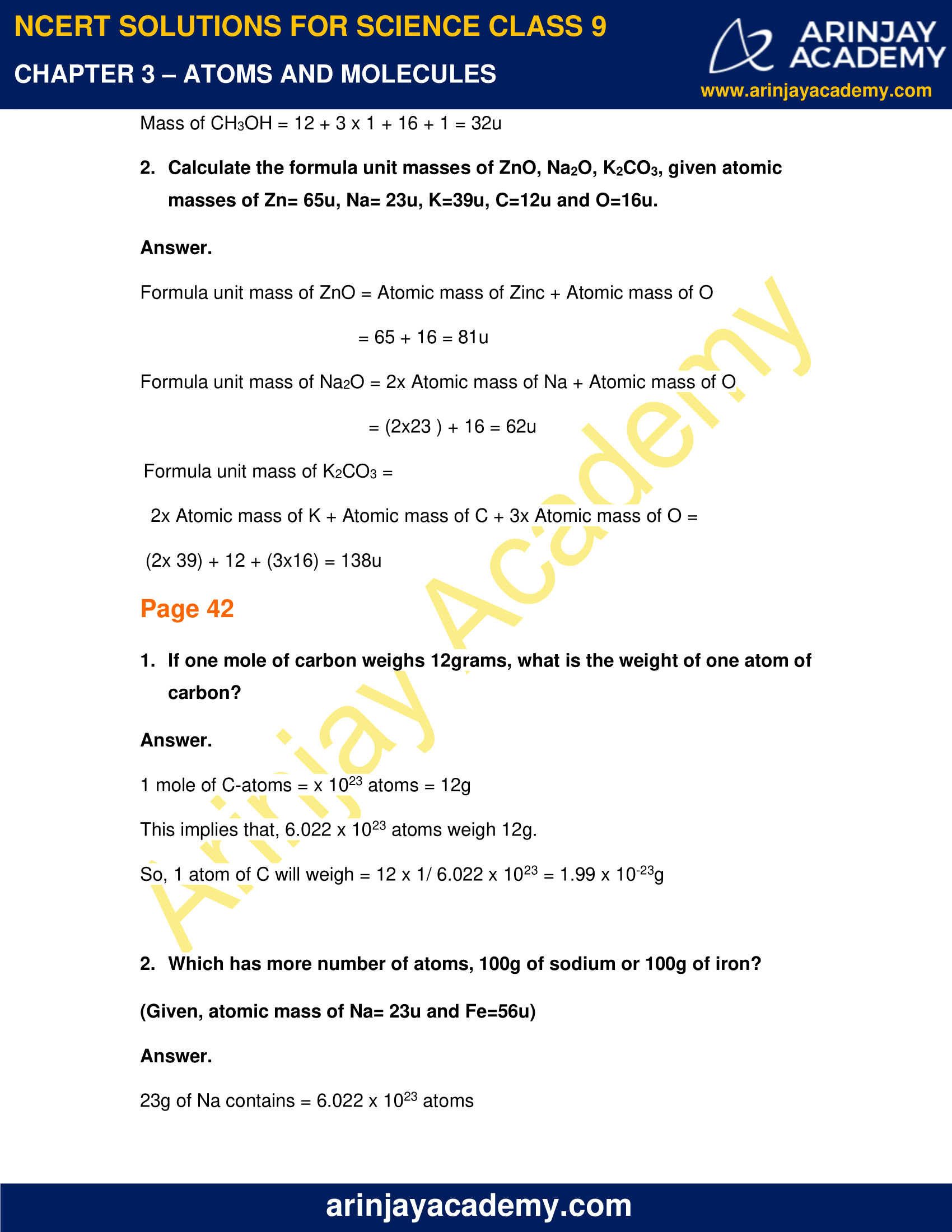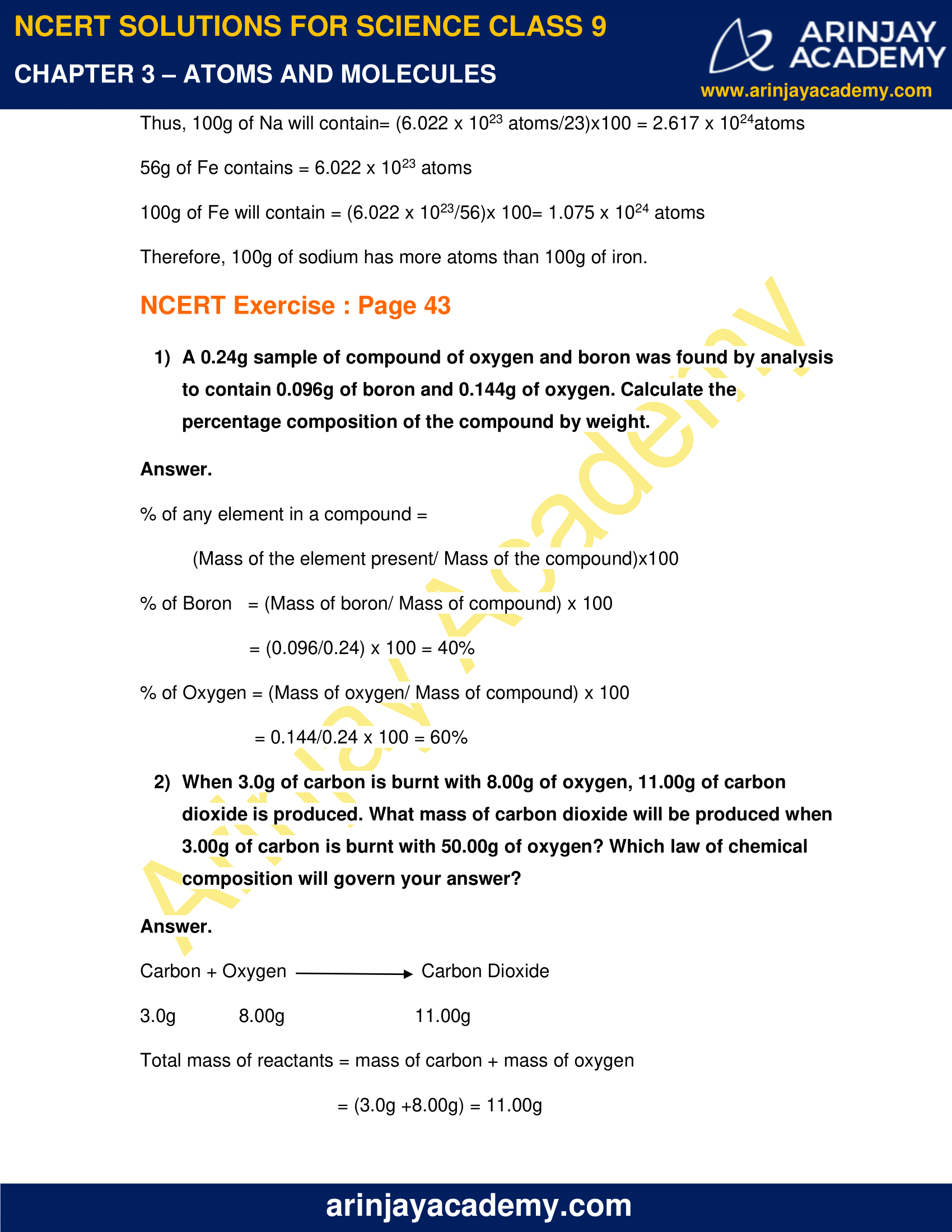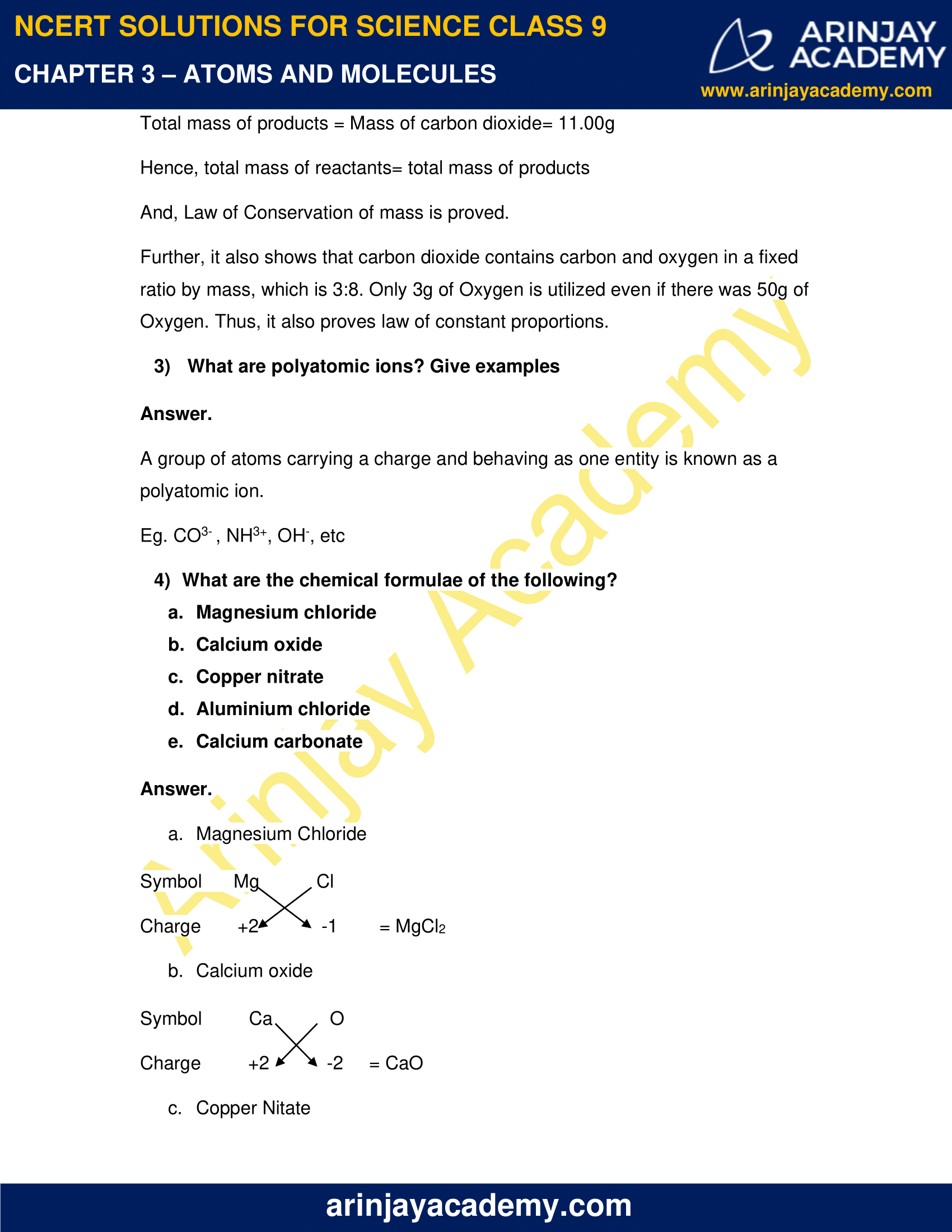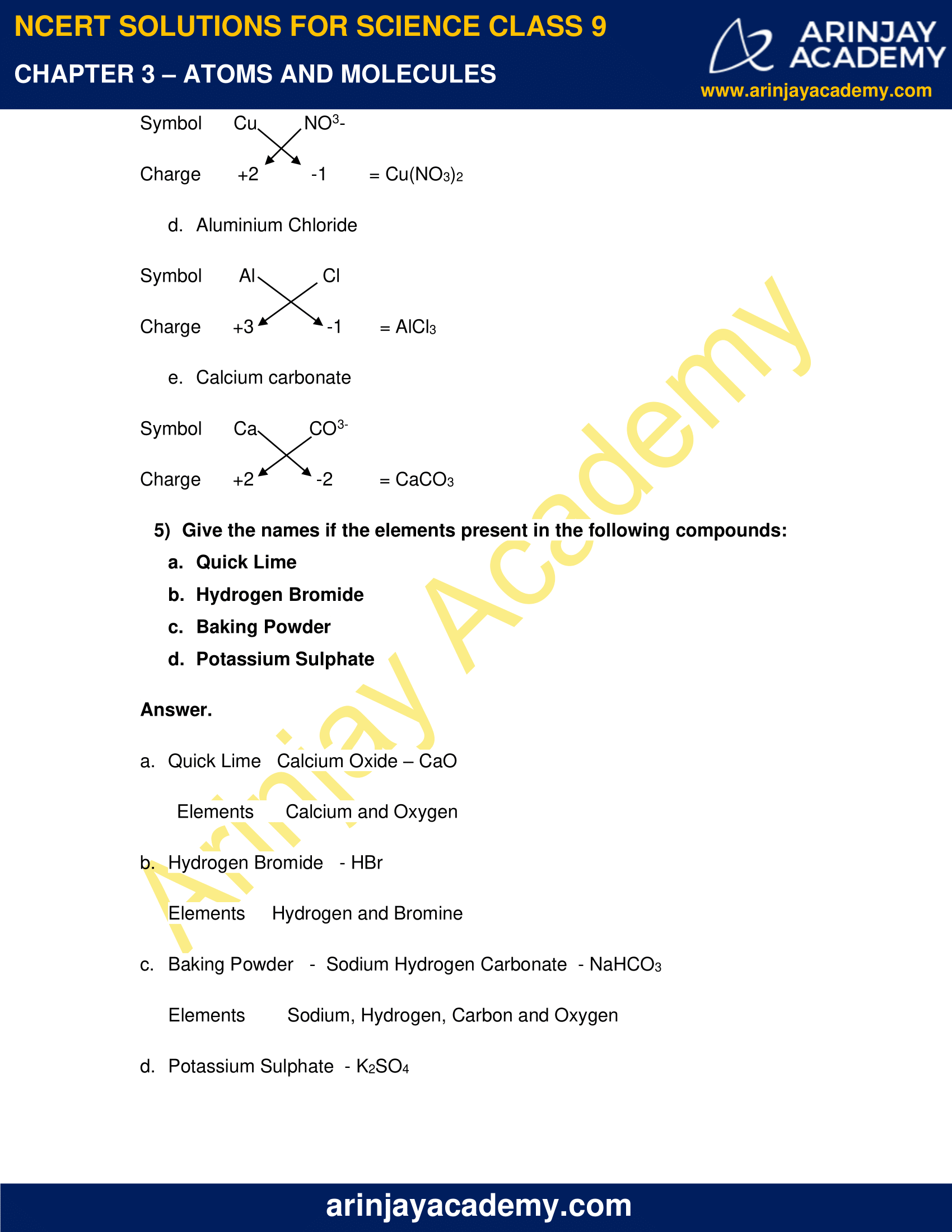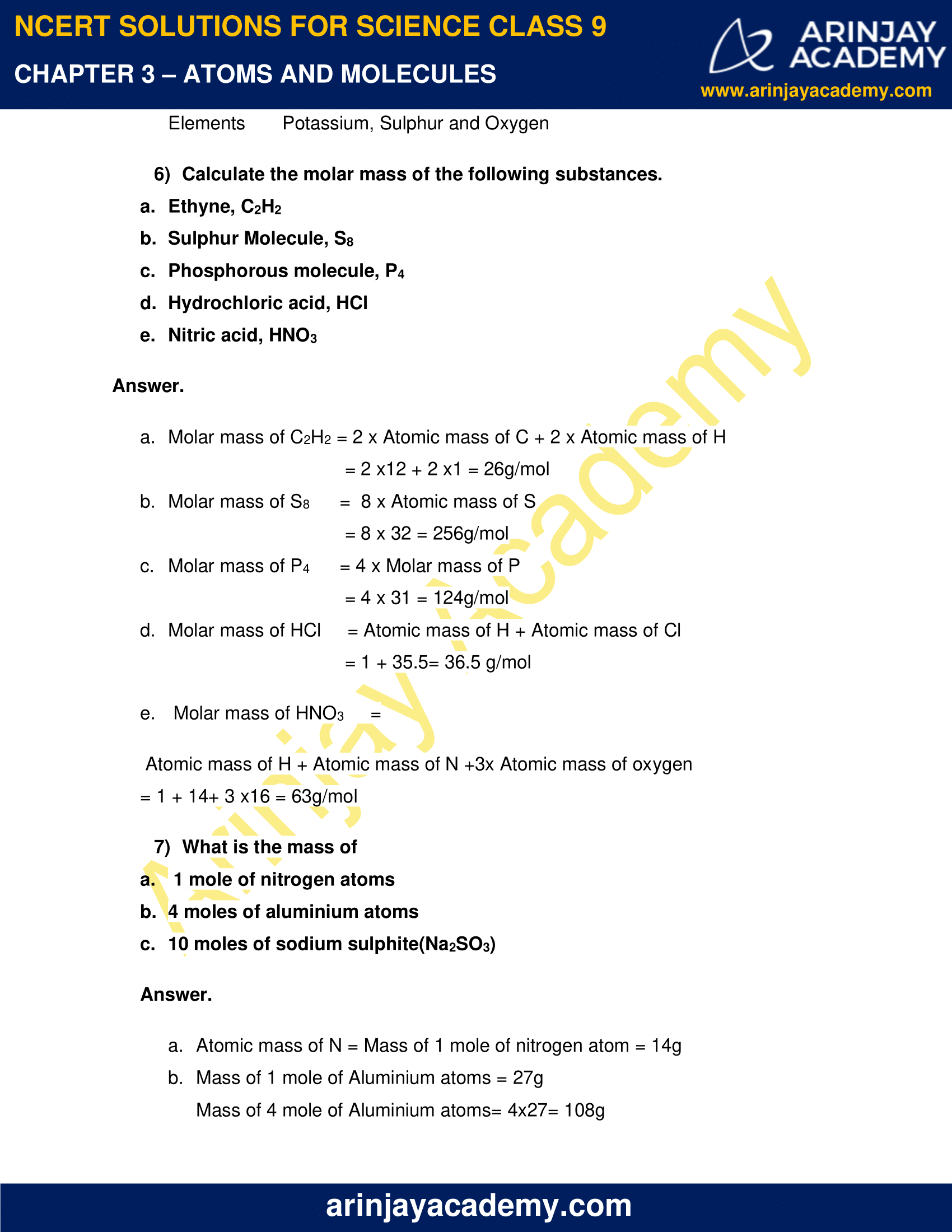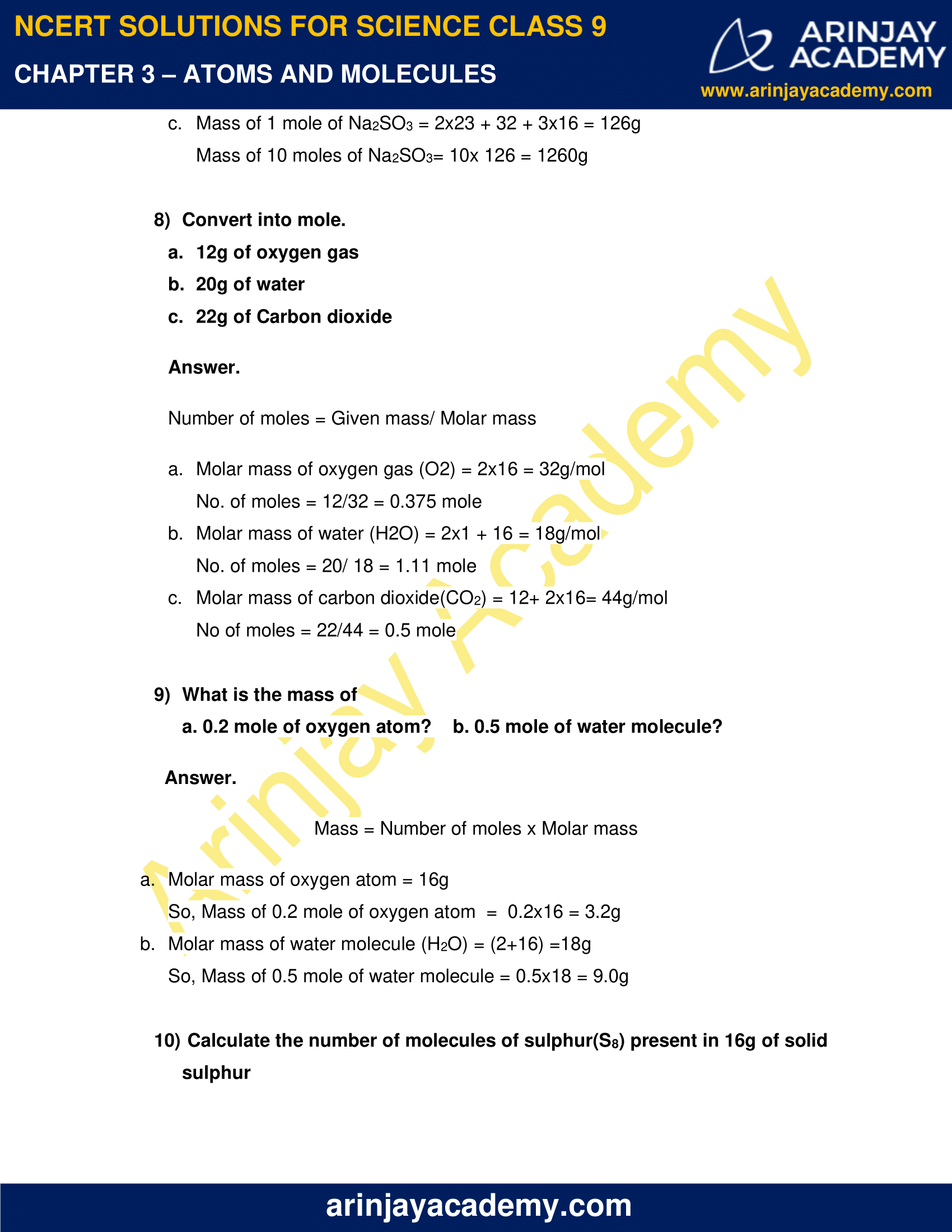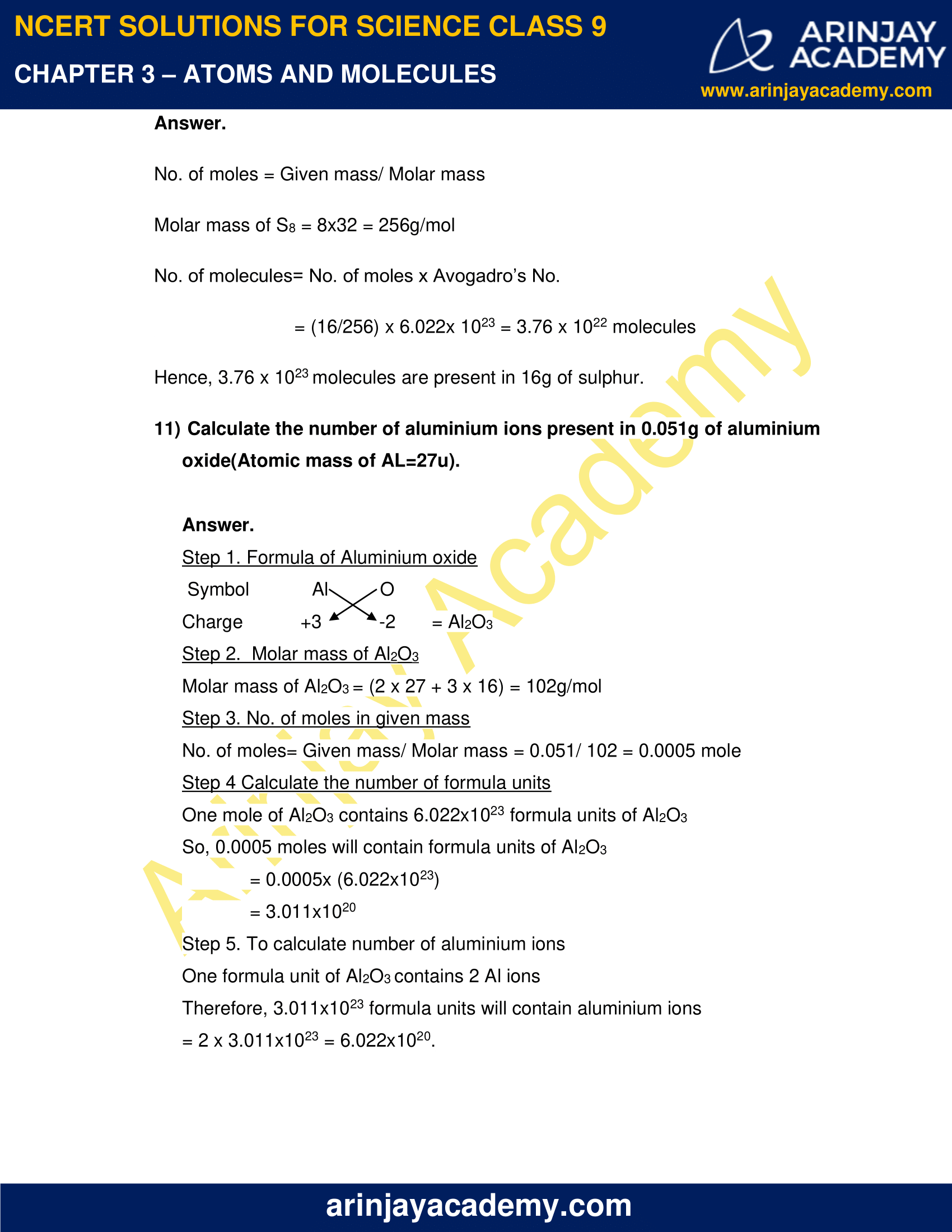### NCERT Solutions for Class 9 Science Chapter 3 – Atoms and Molecules – NCERT Exercises

NCERT Solutions for Class 9 Science Chapter 3 – Atoms and Molecules, Exercises includes all in text and exercise solved questions which helps you to understand the topic covered in Chapter 3 Atoms and Molecules, in a better manner to help you to score good marks in your examinations.

### NCERT Solutions for Class 9 Science Chapter 3 – Intext Questions – Page 32

1. In a reaction, 5.3g of sodium carbonate reacted with 6g of ethanoic acid. The products were 2.2g of carbon dioxide, 0.9g of water and 8.2g of sodium ethanoate. Show that these observations are in agreement with the law of conservation of mass.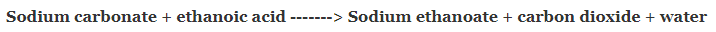Total mass of reactants = Mass of sodium carbonate + mass of ethanoic acid

= 5.3 + 6.0 = 11.3g

Total mass of products = Mass of sodium ethanoate + mass of carbon dioxide + mass of water

= 8.2 + 2.2 + 0.9 = 11.3g

As the total mass of reactants is equal to the total mass of products, therefore the given observation is in agreement with the law of conservation of mass.

2. Hydrogen and oxygen combine in the ratio of 1:8 by mass to form water. What mass of oxygen gas would be required to react completely with 3 g of hydrogen gas?

Since hydrogen and oxygen combine in the ratio of 1:8 by mass,

Mass of hydrogen/ Mass of oxygen = 1:8

Let mass of oxygen required to completely react with 3g of hydrogen be x

Thus, 1/8 = 3/x

so, x = 24g

Therefore, we conclude that 24g of oxygen is required to react with 3g of hydrogen to form water.

3. Which postulate of Dalton’s atomic theory is the result of the law of conservation of mass?

The postulate of Dalton’s atomic theory  which is the result of law of conservation of mass states that, “Atoms are invisible particles which can neither be created nor be destroyed in during a chemical reaction.”

4. Which postulate of Dalton’s atomic theory explains the law of definite proportion?

“The relative number and kinds of atoms are constant in a given compound”, this postulate of Dalton’s atomic theory explains the law of definite proportion.

### NCERT Solutions for Class 9 Science Chapter 3 – Intext Questions – Page 35

1. Define atomic mass unit.

One atomic mass unit (u) is the mass unit equal to exactly 1/12th of the mass of one atom of Carbon-12 isotope. For chemical calculations, the relative atomic masses of all elements have been found with respect to an atom of C-12.

2. Why is it not possible to see an atom with naked eyes.

Atoms are extremely tiny particles, smaller than anything we can imagine. Their radii is measured in terms of nanometers (1nm = 10-9m). Hence they cannot be seen by our naked eyes.

### Page 39

1. Write down the formulae of

i. Sodium oxide
ii. Aluminium chloride
iii. Sodium sulphide
iv. Magnesium hydroxide

The formulae are-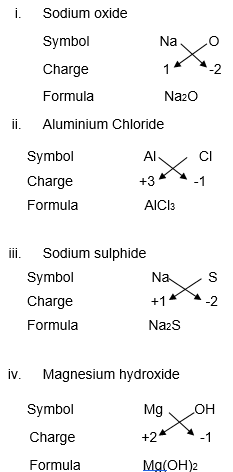2. Write down the names of the compounds represented by the following formulae:

i. Al2(SO4)2
ii. CaCl2
iii. K2SO4
iv. KNO3
v. CaCO3

i. Aluminium sulphate
ii. Calcium chloride
iii. Potassium sulphate
iv. Potassium nitrate
v. Calcium carbonate

3. What is mean by the term chemical formula?

A chemical formula is a simple representation of a chemical compound with the help of symbols and valencies (charges) of elements.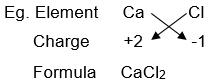4. How many atoms are present in a

i. H2S molecule
ii. PO43-ion

i. In H2S, three atoms are present (i.e. 2 atoms of hydrogen + 1 atom of sulphur)
ii. In PO43-, five atoms are present (i.e.1 atom of phosphorous +4 atoms of oxygen)

### NCERT Solutions for Class 9 Science Chapter 3 – Intext Questions – Page 40

1. Calculate the molecular masses of H2, O2, Cl2, CO2, CH4, C2H6, C2H4, NH3, CH3

For a given molecule,

Molecular mass = Number of atoms * Atomic mass

Therefore,

Mass of H2 = 2 x 1= 2u

Mass of O2 = 2 x 16 = 32u

Mass of Cl2 = 2 x 35.5 = 71u

Mass of CO2 = 12 + 2 x 16 = 44u

Mass of CH4 = 12 + 4 x 1 = 16u

Mass of C2H6 = 2 x 12 + 6 x 1 = 30u

Mass of C2H4 = 2 x12 + 4 x 1= 28u

Mass of NH3 = 14 + 3 x 1 = 17u

Mass of CH3OH = 12 + 3 x 1 + 16 + 1 = 32u

2. Calculate the formula unit masses of ZnO, Na2O, K2CO3, given atomic masses of Zn= 65u, Na= 23u, K=39u, C=12u and O=16u.

Formula unit mass of ZnO

= Atomic mass of Zinc + Atomic mass of O

= 65 + 16 = 81u

Formula unit mass of Na2O

= 2x Atomic mass of Na + Atomic mass of O

= (2×23 ) + 16 = 62u

Formula unit mass of K2CO3

= 2x Atomic mass of K + Atomic mass of C + 3x Atomic mass of O =

(2x 39) + 12 + (3×16) = 138u

### Page 42

1. If one mole of carbon weighs 12 grams, what is the weight of one atom of carbon?

1 mole of C-atoms = 6.022 x 1023 atoms = 12g

This implies that, 6.022 x 1023 atoms weigh 12g.

So, 1 atom of C will weigh = 12 x 1/ 6.022 x 1023 = 1.99 x 10-23g

2. Which has more number of atoms, 100g of sodium or 100g of iron?

(Given, atomic mass of Na= 23u and Fe=56u)

23g of Na contains = 6.022 x 1023 atoms

Thus, 100g of Na will contain= (6.022 x 1023 atoms/23)x100 = 2.617 x 1024atoms

56g of Fe contains = 6.022 x 1023 atoms

100g of Fe will contain = (6.022 x 1023/56)x 100= 1.075 x 1024 atoms

Therefore, 100g of sodium has more atoms than 100g of iron.

### NCERT Solutions for Class 9 Science Chapter 3 – NCERT Exercise – Page 43

1. A 0.24g sample of compound of oxygen and boron was found by analysis to contain 0.096g of boron and 0.144g of oxygen. Calculate the percentage composition of the compound by weight.

% of any element in a compound =

(Mass of the element present/ Mass of the compound)x100

% of Boron   = (Mass of boron/ Mass of compound) x 100

= (0.096/0.24) x 100 = 40%

% of Oxygen = (Mass of oxygen/ Mass of compound) x 100

= 0.144/0.24 x 100 = 60%

2. When 3.0g of carbon is burnt with 8.00g of oxygen, 11.00g of carbon dioxide is produced. What mass of carbon dioxide will be produced when 3.00g of carbon is burnt with 50.00g of oxygen? Which law of chemical composition will govern your answer?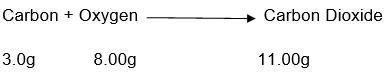Total mass of reactants = mass of carbon + mass of oxygen

= (3.0g +8.00g) = 11.00g

Total mass of products = Mass of carbon dioxide= 11.00g

Hence, total mass of reactants= total mass of products

And, Law of Conservation of mass is proved.

Further, it also shows that carbon dioxide contains carbon and oxygen in a fixed ratio by mass, which is 3:8. Only 3g of Oxygen is utilized even if there was 50g of Oxygen. Thus, it also proves law of constant proportions.

3. What are polyatomic ions? Give examples

A group of atoms carrying a charge and  behaving as one entity is known as a polyatomic ion.

Eg. CO3- , NH3+, OH, etc

4. What are the chemical formulae of the following?

a) Magnesium chloride
b) Calcium oxide
c) Copper nitrate
d) Aluminium chloride
e) Calcium carbonate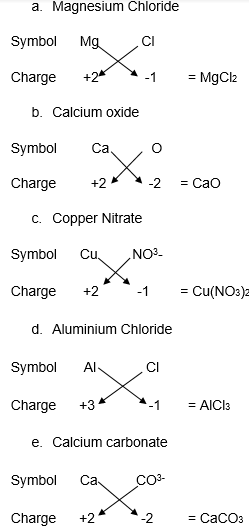5. Give the names if the elements present in the following compounds:

a) Quick Lime
b) Hydrogen Bromide
c) Baking Powder
d) Potassium Sulphate

a) Quick Lime Calcium Oxide – CaO

Elements – Calcium and Oxygen

b) Hydrogen Bromide – HBr

Elements – Hydrogen and Bromine

c) Baking Powder –  Sodium Hydrogen Carbonate  – NaHCO3

Elements – Sodium, Hydrogen, Carbon and Oxygen

d) Potassium Sulphate – K2SO4

Elements – Potassium, Sulphur and Oxygen

6. Calculate the molar mass of the following substances.

a) Ethyne, C2H2
b) Sulphur Molecule, S8
c) Phosphorous molecule, P4
d) Hydrochloric acid, HCl
e) Nitric acid, HNO3

a) Molar mass of C2H2 = 2 x Atomic mass of C + 2 x Atomic mass of H

= 2 x12 + 2 x1 = 26g/mol

b) Molar mass of S8 =  8 x Atomic mass of S

= 8 x 32 = 256g/mol

c) Molar mass of P4 = 4 x Molar mass of P

= 4 x 31 = 124g/mol

d) Molar mass of HCl = Atomic mass of H + Atomic mass of Cl

= 1 + 35.5= 36.5 g/mol

e) Molar mass of HNO3 =Atomic mass of H + Atomic mass of N +3x Atomic mass of oxygen

= 1 + 14+ 3 x16 = 63g/mol

7. What is the mass of

a) 1 mole of nitrogen atoms

b) 4 moles of aluminium atoms

c) 10 moles of sodium sulphite(Na2SO3)

a) Atomic mass of N = Mass of 1 mole of nitrogen atom = 14g

b) Mass of 1 mole of Aluminium atoms = 27g

Mass of 4 mole of Aluminium atoms= 4×27= 108g

c) Mass of 1 mole of Na2SO3 = 2×23 + 32 + 3×16 = 126g

Mass of 10 moles of Na2SO3= 10x 126 = 1260g

8. Convert into mole.

a) 12g of oxygen gas
b) 20g of water
c) 22g of Carbon dioxide

Number of moles = Given mass/ Molar mass

a. Molar mass of oxygen gas (O2) = 2×16 = 32g/mol

No. of moles = 12/32 = 0.375 mole

b. Molar mass of water (H2O) = 2×1 + 16 = 18g/mol

No. of moles = 20/ 18 = 1.11 mole

c. Molar mass of carbon dioxide(CO2) = 12+ 2×16= 44g/mol

No of moles = 22/44 = 0.5 mole

9. What is the mass of

a) 0.2 mole of oxygen atom?

b) 0.5 mole of water molecule?

Mass = Number of moles x Molar mass

a) Molar mass of oxygen atom = 16g

So, Mass of 0.2 mole of oxygen atom  =  0.2×16 = 3.2g

b) Molar mass of water molecule (H2O) = (2+16) =18g

So, Mass of 0.5 mole of water molecule = 0.5×18 = 9.0g

10. Calculate the number of molecules of sulphur(S8) present in 16g of solid sulphur

No. of moles = Given mass/ Molar mass

Molar mass of S8 = 8×32 = 256g/mol

No. of molecules= No. of moles x Avogadro’s No.

= (16/256) x 6.022x 1023 = 3.76 x 1022 molecules

Hence, 3.76 x 1023 molecules are present in 16g of sulphur.

11. Calculate the number of aluminium ions present in 0.051g of aluminium oxide(Atomic mass of AL=27u).

Step 1. Formula of Aluminium oxide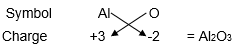Step 2.  Molar mass of Al2O3

Molar mass of Al2O3 = (2 x 27 + 3 x 16) = 102g/mol

Step 3. No. of moles in given mass

No. of moles= Given mass/ Molar mass = 0.051/ 102 = 0.0005 mole

Step 4 Calculate the number of formula units

One mole of Al2O3 contains 6.022×1023 formula units of Al2O3

So, 0.0005 moles will contain formula units of Al2O3

= 0.0005x (6.022×1023)

= 3.011×1020

Step 5. To calculate number of aluminium ions

One formula unit of Al2O3 contains 2 Al ions

Therefore, 3.011×1023 formula units will contain aluminium ions

= 2 x 3.011×1023 = 6.022×1020.

### Topics Covered in Atoms and Molecules Class 9 Science

1. Laws of Chemical Combination
2. What is an Atom
3. What is a Molecule
4. Writing Chemical Formulae
• Formulae of Simple Compounds
5. Molecular Mass and Mole Concept
• Molecular Mass
• Mole Concept